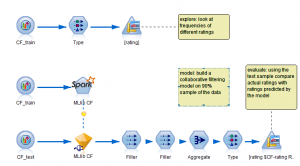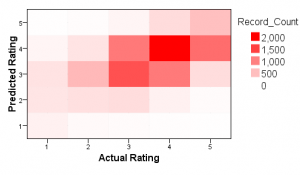# Watson Studio

View Only

### Coding a Python/Spark Modeler Extension for Collaborative Filtering

#### By Archive User posted Mon March 07, 2016 04:58 PM

Introduction

IBM SPSS Modeler 17.1 introduces the ability to build extension nodes written in the Python2 language and leveraging Apache Spark via the Python for Spark (pyspark) API. This feature is introduced in an earlier post. To run extension nodes built in python, Modeler needs to be connected to a Hadoop Cluster in which Spark is enabled, via the Analytic Server service.

So, Python for Spark based extension nodes can leverage the data transformation features available in Spark, and also the model building and scoring available in Spark's Machine Learning Library (MLlib).

In this article we'll look at the code used in a Modeler extension node which allow modeler streams to leverage Spark's Collaborative Filtering algorithm to build a simple recommender system.

What is Collaborative Filtering?

Collaborative Filtering is a technique which models the preferences of users for items. An item could for example be a song, film or book - in fact any thing which a user could give some kind of rating (or even just choose to buy). The model is constructed by analyzing historical data which describes, for pairs of user and item, the rating given by that user to that item. Usually, the number of users and items is very large (this can be a big data problem) although the data is sparse (only a few possible combinations of user and item have a rating).

Spark MLlib contains the Alternating Least Squares algorithm which can analyze this data to model user tastes, and also then apply that model to predict the rating that a user would give for a product that they have not yet rated. The model may therefore be used to find new items that user might like (and potentially, such items can then be recommended to the user).

Try it!

To install and try the Collaborative Filtering extension node, you'll need to be running SPSS Modeler 17.1 or later, connected to SPSS Analytic Server and a Hadoop Cluster with Spark and Python installed. Here are the steps:

https://github.com/IBMPredictiveAnalytics/MLlib_CF.

2. Once you have installed the node, you can run a simple test using training and test data derived from the 100Krow dataset from the movielens project. Create Analytic Server data sources called CF_test (using downloaded file CF_Test.csv) and CF_train (using downloaded file CF_Train.csv).

3. Finally download a sample stream and load it into Modeler. Follow the instructions in comments pasted into the stream. The stream can be used to train a collaborative filtering model from the 90k rows in CF_Train dataset and then test its accuracy by comparing actual versus predicted ratings on the CF_Test dataset.

The sample stream:Sample results - comparing predicted versus actual ratings on the CF_test dataset:In the remainder of this post, we take a look at the code inside an SPSS Modeler extension node that integrates with this collaborative filtering algorithm. Unlike most modeler model building nodes, we won't aim to store the model's content in a model nugget which is returned to modeler client when the model is built. This is because the size of the model will increase with the size of the training dataset and may become too large to transfer over the network. Instead as one of our build parameters we will specify a path on the Hadoop Cluster's Filesystem (HDFS) where the model will be stored, and include that path in the model nugget.

Python Code for Model Building

Here is the script for building an ALS model that is embedded into the extension node.

First, lets import the various python libraries we will need and get parameters for the script. Note that text strings of the form %%value%% are substituted from the UI controls we've defined in the node's user interface.

`from pyspark.context import SparkContext`
`from pyspark.sql.context import SQLContext`
`from pyspark.mllib.recommendation import ALS, Rating`

`import spss.pyspark.runtime`
`import json`

`user_field = '%%user_field%%'`
`item_field = '%%item_field%%'`
`rating_field = '%%rating_field%%'`
`model_path = '%%model_path%%'`
`rank = %%rank%%`
`iterations = %%iterations%%`
`lmbda= %%lmbda%%`
`blocks = %%blocks%%`
`random_seed = long(%%random_seed%%)`

Next we obtain the SparkContext (sc) and input data in the form of a Spark DataFrame (df). The DataFrame is a standard API for representing rows and columns of data with well defined types for each column. We work out the index into each row where we will obtain the values for user, item and rating.

`ascontext = spss.pyspark.runtime.getContext()`
`sc = ascontext.getSparkContext()`
`df = ascontext.getSparkInputData()`
`count = df.count()`

`schema = df.dtypes[:]`
`user_index = [col for col in schema].index(user_field)`
`item_index = [col for col in schema].index(item_field)`
`rating_index = [col for col in schema].index(rating_field)`

Now its time to convert from the DataFrame to an resilient distributed dataset (RDD) of Rating objects which the ALS algorithm will accept as input, and then build the model with the appropriate parameters.

`ratings = df.map(lambda row:`
` Rating(`
` int(row[user_index]),`
` int(row[item_index]),`
` float(row[rating_index])))`

`model = ALS.train(ratings, rank, iterations, lmbda, blocks, seed=random_seed)`

Finally we need to persist the model to HDFS so it can later be used for scoring. We also return a JSON encoded object containing the number of records in the training data, to be incorporated into the model nugget.

`model.save(sc, model_path)`

`path=ascontext.createTemporaryFolder()`
`sc.parallelize([json.dumps({"training_data_set_size":count})]).saveAsTextFile(path)`
`ascontext.setModelContentFromPath("model",path)`

Being able to build a Colaborative Filtering model is the first step, but next we need to work out how we could use the model as part of a recommender system. In the next section we will create an accompanying script for our extension node which explains how the model will be scored.

Python Code for Model Scoring

One way of using the model we have built is to score it, so that we pass in rows containing a user and an item, we then use the model predict the rating that the user might assign to the item, and we will add an extra column to our dataset containing these predicted scores. The python script to score the model looks like this. First we start with imports and retrieve the parameters we will need.

`from pyspark.context import SparkContext`
`from pyspark.sql.context import SQLContext`
`from pyspark.mllib.recommendation import ALS, MatrixFactorizationModel, Rating`
`import spss.pyspark.runtime`
`from pyspark.sql.functions import udf`
`from pyspark.sql.types import StringType, IntegerType, DoubleType, StructField, StructType`
`import sys`

`user_field = '%%user_field%%'`
`item_field = '%%item_field%%'`
`rating_field = '%%rating_field%%'`
`model_path = '%%model_path%%'`

In the next block of code, we retrieve the SPSS and Spark API objects and get the input dataframe. We compute the output data model for our model nugget as the input data model, plus a new field which will be our predicted rating. The name of the new field is formed by prepending the string \$CF- to the name of the rating field from the training data.

`ascontext = spss.pyspark.runtime.getContext()`
`sc = ascontext.getSparkContext()`
`sqlCtx = ascontext.getSparkSQLContext()`

`prediction_field = "\$CF-" + rating_field`
`_schema = ascontext.getSparkInputSchema()`
`_schema.fields.append(StructField(prediction_field, DoubleType(), nullable=True))`
`ascontext.setSparkOutputSchema(_schema)`

If our script is invoked only in order to discover the output data model, we exit here...

`if ascontext.isComputeDataModelOnly():`
` sys.exit(0)`

...otherwise we now read the data and do the actual processing. We take the input data and transform it to obtain an RDD containing (user,item) tuples.

`df = ascontext.getSparkInputData()`
`schema = df.dtypes[:]`
`user_index = [col for col in schema].index(user_field)`
`item_index = [col for col in schema].index(item_field)`
`rating_index = [col for col in schema].index(rating_field)`
`scoredata = df.map(lambda l: (int(l[user_index]), int(l[item_index])))`

Next we load the model from HDFS and invoke its predictAll method to obtain predicted rating for each (user,item) tuple, transforming the result back to a DataFrame.

`model = MatrixFactorizationModel.load(sc, model_path)`
`predictions = model.predictAll(scoredata).map(lambda x: (x, x,x ))`
`predictdf = sqlCtx.createDataFrame(predictions,[user_field, item_field,prediction_field])`

`concat1 = udf(lambda a,b: str(a)+"_"+str(b))`
`right = predictdf.withColumn("key",concat1(predictdf[user_field],predictdf[item_field]))`
` .select('key',prediction_field) `
`left = df.withColumn("key",concat1(df[user_field],df[item_field]))`

`outnames = [col for col in schema] + [prediction_field]`
`outdf = left.join(right,left['key'] == right['key'], 'inner').select(*outnames)`

`ascontext.setSparkOutputData(outdf)`

Next Steps

Try experimenting with much larger movielens datasets. Visit the Predictive Analytics Gallery to find other python-based modeler extensions. Try creating your own extension nodes based on Python, Spark and MLlib and share them with the community!

F. Maxwell Harper and Joseph A. Konstan. 2015. The MovieLens Datasets:
History and Context. ACM Transactions on Interactive Intelligent
Systems (TiiS) 5, 4, Article 19 (December 2015), 19 pages.
DOI=http://dx.doi.org/10.1145/2827872

IBM SPSS Modeler 17.1 Extensions Manual ftp://public.dhe.ibm.com/software/analytics/spss/documentation/modeler/17.1/en/ModelerRnodes.pdf

Predictive Analytics Gallery http://ibmpredictiveanalytics.github.io/

Alternating Least Squares for Collaborative Filtering http://bugra.github.io/work/notes/2014-04-19/alternating-least-squares-method-for-collaborative-filtering/

#Spark
#SPSSModeler
#Programmability
#python
#Algorithms
5 views